# 同步Synchronization

## synchronized关键字锁对象（引用变量）

``````Object o = new Object();

synchronized (o) { // Get the lock of Object o
// Code guarded by the lock
}
``````

## synchronized+this关键字锁代码块

``````// Get the lock of the object this code belongs to
synchronized (this) {
// Code guarded by the lock
}
``````

## synchronized 锁方法

``````public synchronize void method() {
// Code guarded by the lock
}
``````

``````public void method() {
synchronized (this) {
// Code guarded by the lock
}
}
``````

## synchronized 锁静态static方法

``````public void method() {
synchronized (this) {
// Code guarded by the lock
}
}
``````

``````class MyClass {
synchronized static void method() {
/** .. */
}
}
``````
• 示例
``````static int n = 0;
static int n1=0;
n++;
System.out.println(n);
}
n1++;
System.out.println(n1+"synchronized");
}
public static void main(String[] args) {
Runnable r = () -> add();
for(int i = 0; i < 4; i++) {
service.execute(r);
service.execute(r1);
}
service.shutdown();
}
``````# Atomic Classes原子类

java.concurrent.atomic包含执行原子操作的类，这些操作在单个步骤中执行，不受多线程的干预。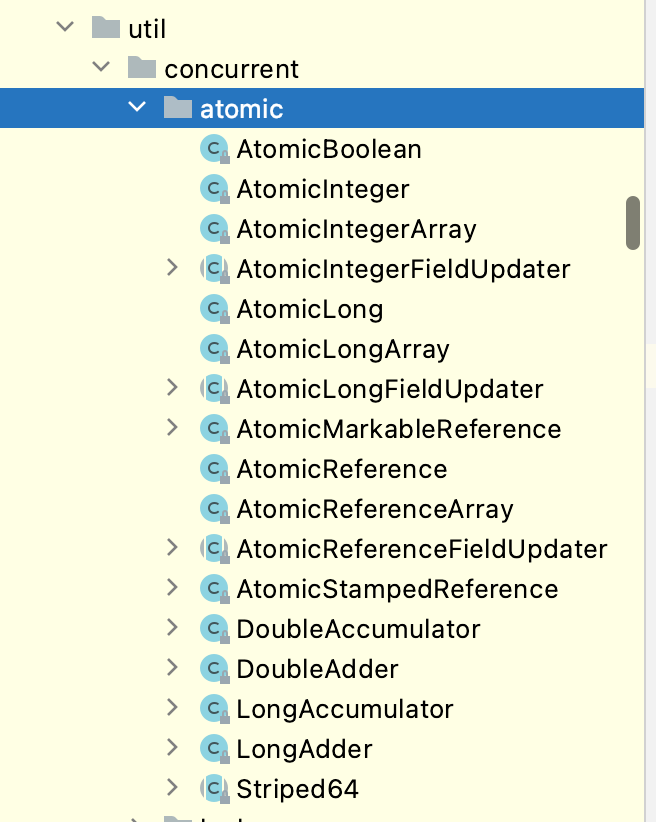• 例如AtomicInteger类
``````static AtomicInteger n2 = new AtomicInteger(0);
System.out.println(n2.incrementAndGet()+"Atomic");
}
public static void main(String[] args) {
Runnable r = () -> add();
Runnable r1 = () -> add1();
Runnable r2 = () -> add2();
for (int i = 0; i < 4; i++) {
service.execute(r);
service.execute(r1);
service.execute(r2);
}
service.shutdown();
``````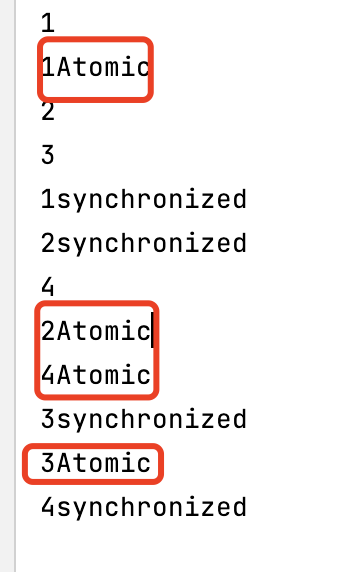# 并发集合

java.util.concurrent包提供了一些线程安全的类，等价于java.util的集合类。不需要显式地同步操作，且性能优于synchronized关键字

``````Map<String, Integer> map = new HashMap<>();
...
void putIfNew(String key, Integer val) {
if(map.get(key) == null) {
map.put(key, val);
}
}
``````

``````Map<String, Integer> map = new ConcurrentHashMap<>();
...
map.putIfAbsent(key, val);
``````

Java的JUC并发包提供了许多并发集合，根据它们实现的接口来对它们进行分类:

• BlockingQueue
• BlockingDeque
• ConcurrentMap
• ConcurrentNavigableMap
由于这些接口是从java.util.Collection接口扩展而来的，它们继承了我们已经知道的方法，因此在JUC接口中这里您只能找到支持并发的添加方法。对于列表，您可以使用类copyonwriterraylist。

## BlockingQueue接口

• 插入
``````void put(E e)
boolean offer(E e, long timeout, TimeUnit unit)
``````
• 移除
``````E take()
E poll(long timeout, TimeUnit unit)
``````

### 实现类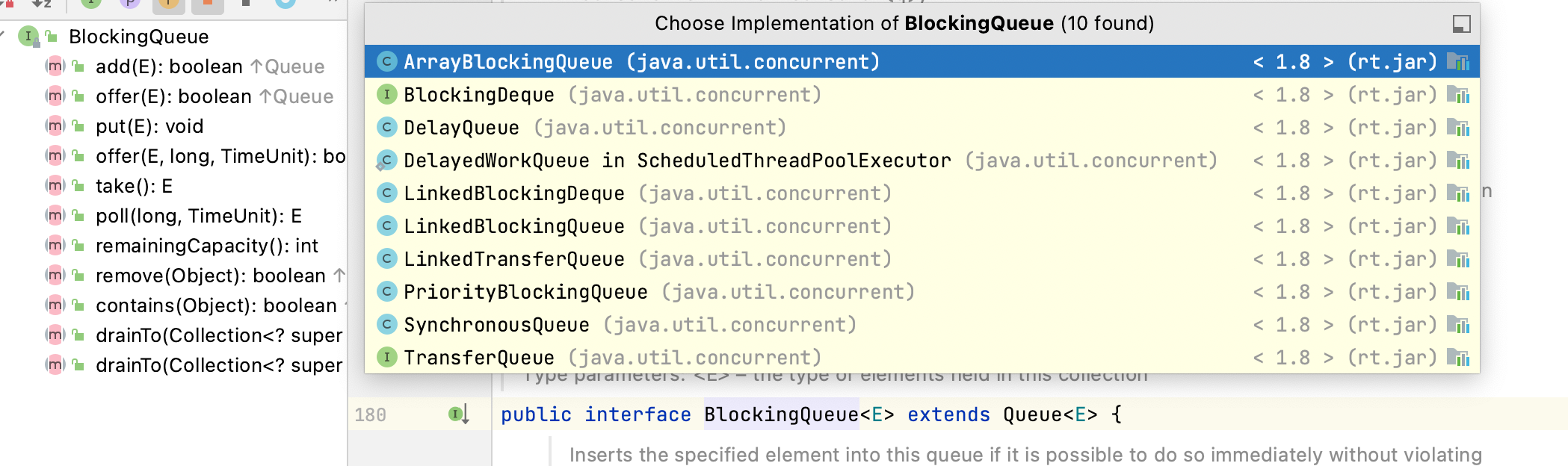• ArrayBlockingQueue
由数组支持的有界阻塞队列
• DelayQueue
无限制的阻塞队列元素，其中一个元素只能在其延迟到期后才能使用
基于链接节点的可选有界阻塞队列
基于连接节点
• PriorityBlockingQueue
无界阻塞队列
• SynchronousQueue
阻塞队列，其中每个插入操作必须等待另一个线程的相应删除操作，反之亦然

## ConcurrentMap接口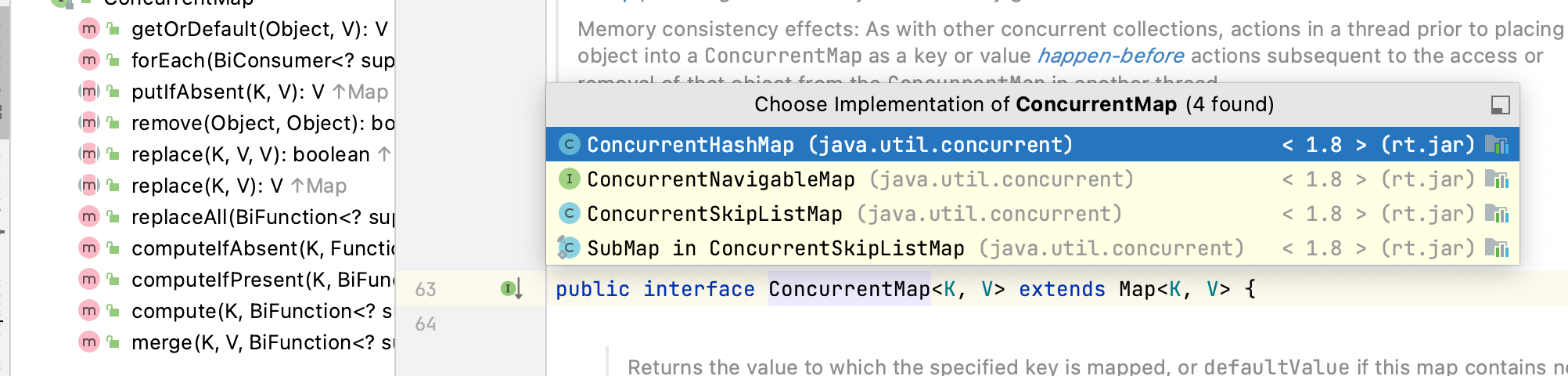### 默认default方法

• 原子地使用 BiFunction 计算指定键的值
``````V compute(K key, BiFunction<? super K,? super V,? extends V> remappingFunction)
``````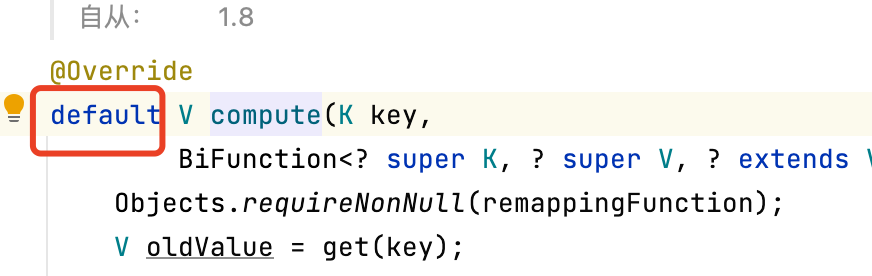• 只有当k不存在于映射中时，原子地计算其值
``````V computeIfAbsent(K key, Function<? super K,? extends V> mappingFunction)
``````
• 只有键出现在映射中时，才能原子计算它的值
``````V computeIfPresent(K key, BiFunction<? super K,? super V,? extends V> remappingFunction)
``````
• 返回键的值，如果键不存在，则返回默认值
``````V getOrDefault(Object key, V defaultValue)
``````

## ConcurrentNavigableMap接口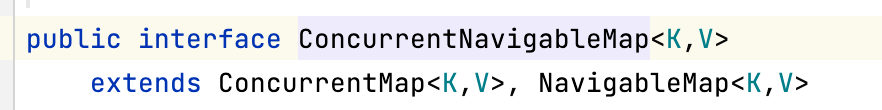## CopyOnWriteArrayList类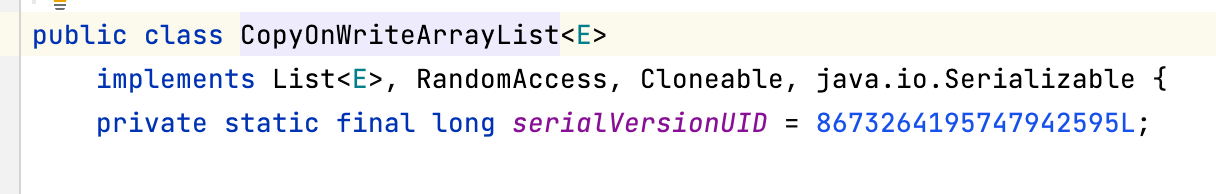• 列表的最后一个元素在每次迭代中都会改变，但原始值仍在迭代器中打印。迭代器使用的是未修改的列表副本，所以值虽然改变但迭代里面的也是打印出的原始值
``````List<Integer> list = new CopyOnWriteArrayList<>();
Iterator<Integer> it = list.iterator();
while(it.hasNext()) {
int i = it.next();
System.out.print(i + " ");
// No exception thrown
list.set(list.size() -1, i * 10);
// it.remove(); throws an exception
}
System.out.println(list);
``````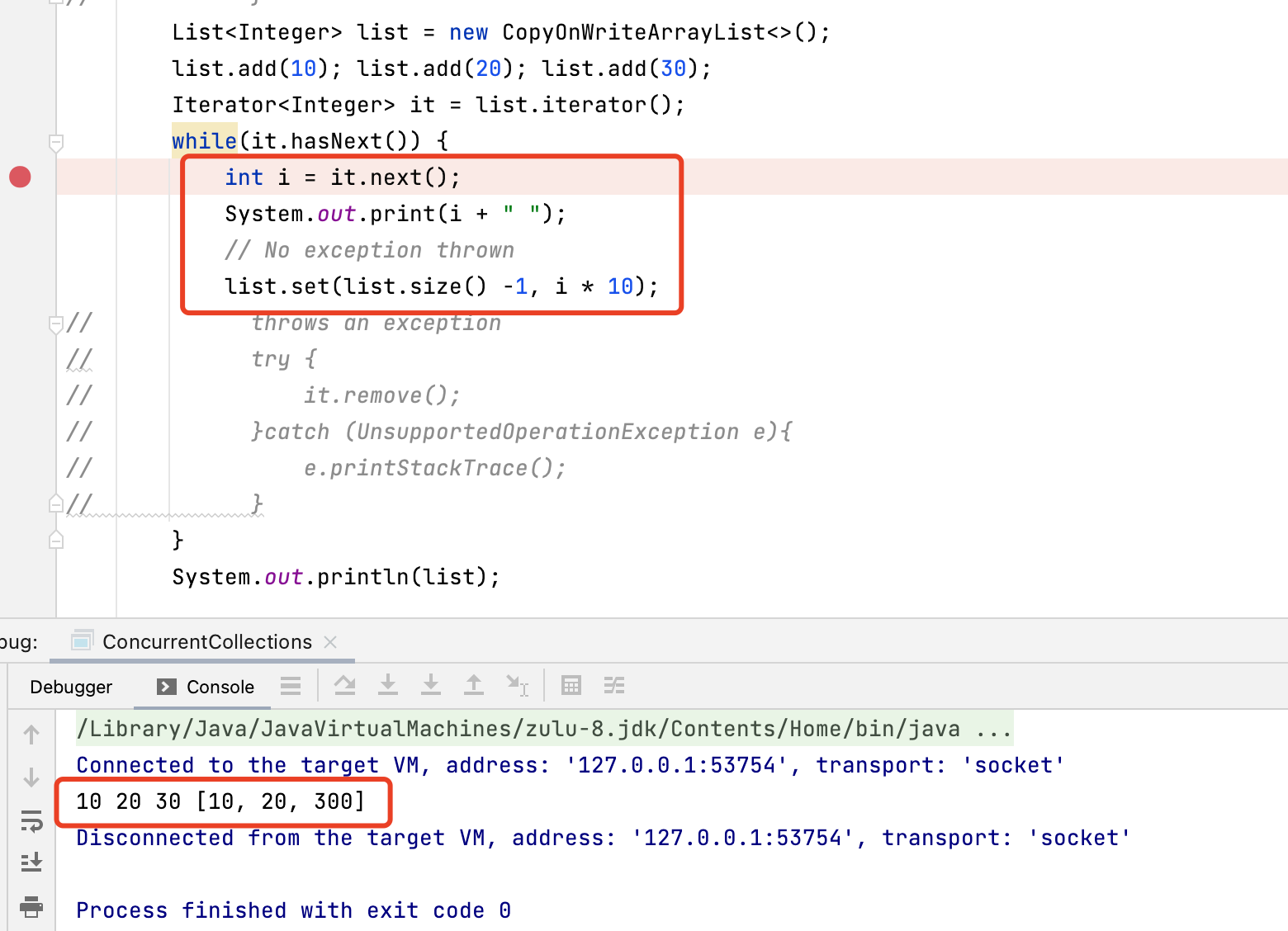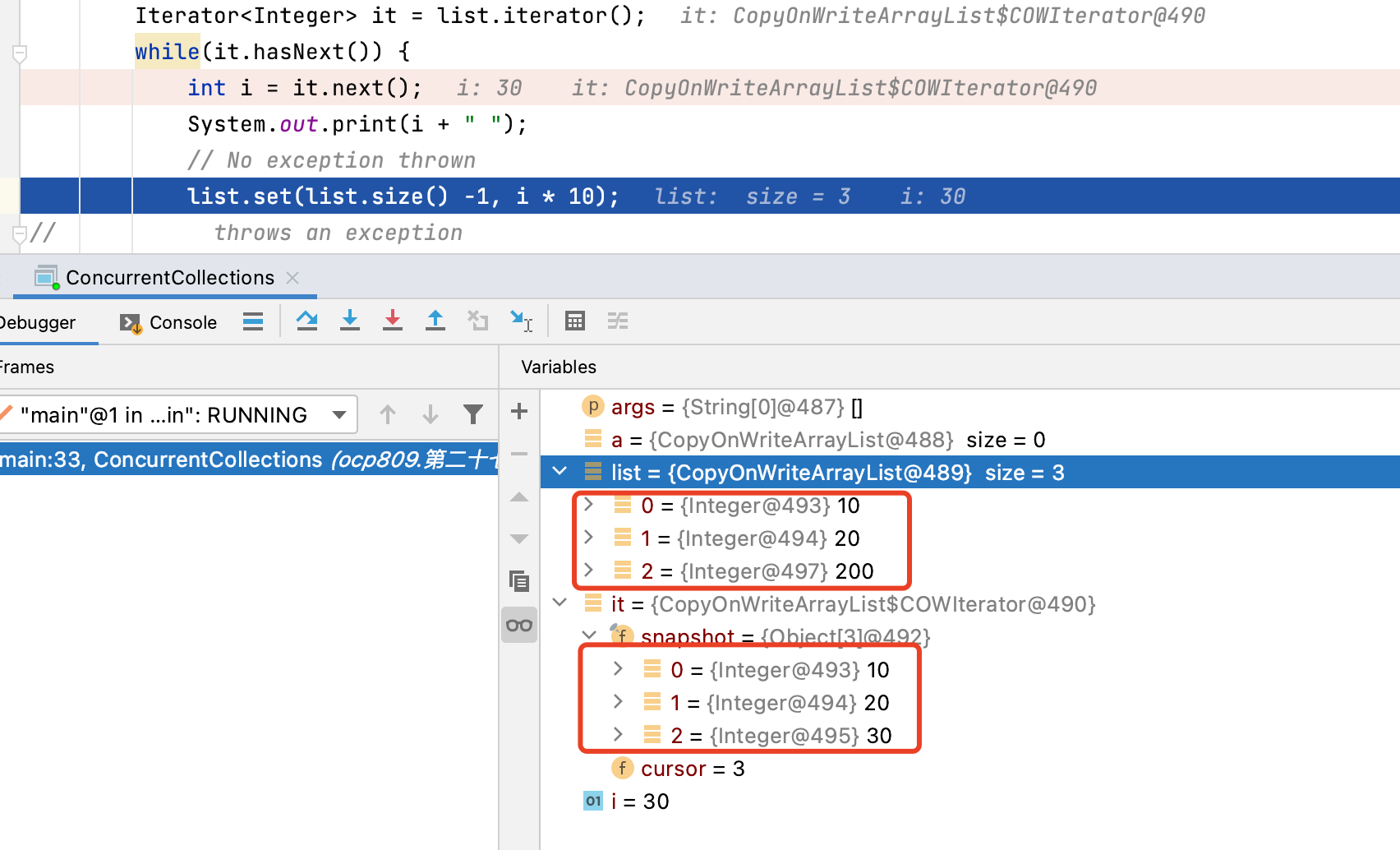• 使用 copyonwriearraylist，对读操作没有锁定，因此这个操作更快。出于这个原因，copyonwriearraylist 在更新和插入很少、并发读取很多的情况下非常有用。

## Collections工具类包装同步普通集合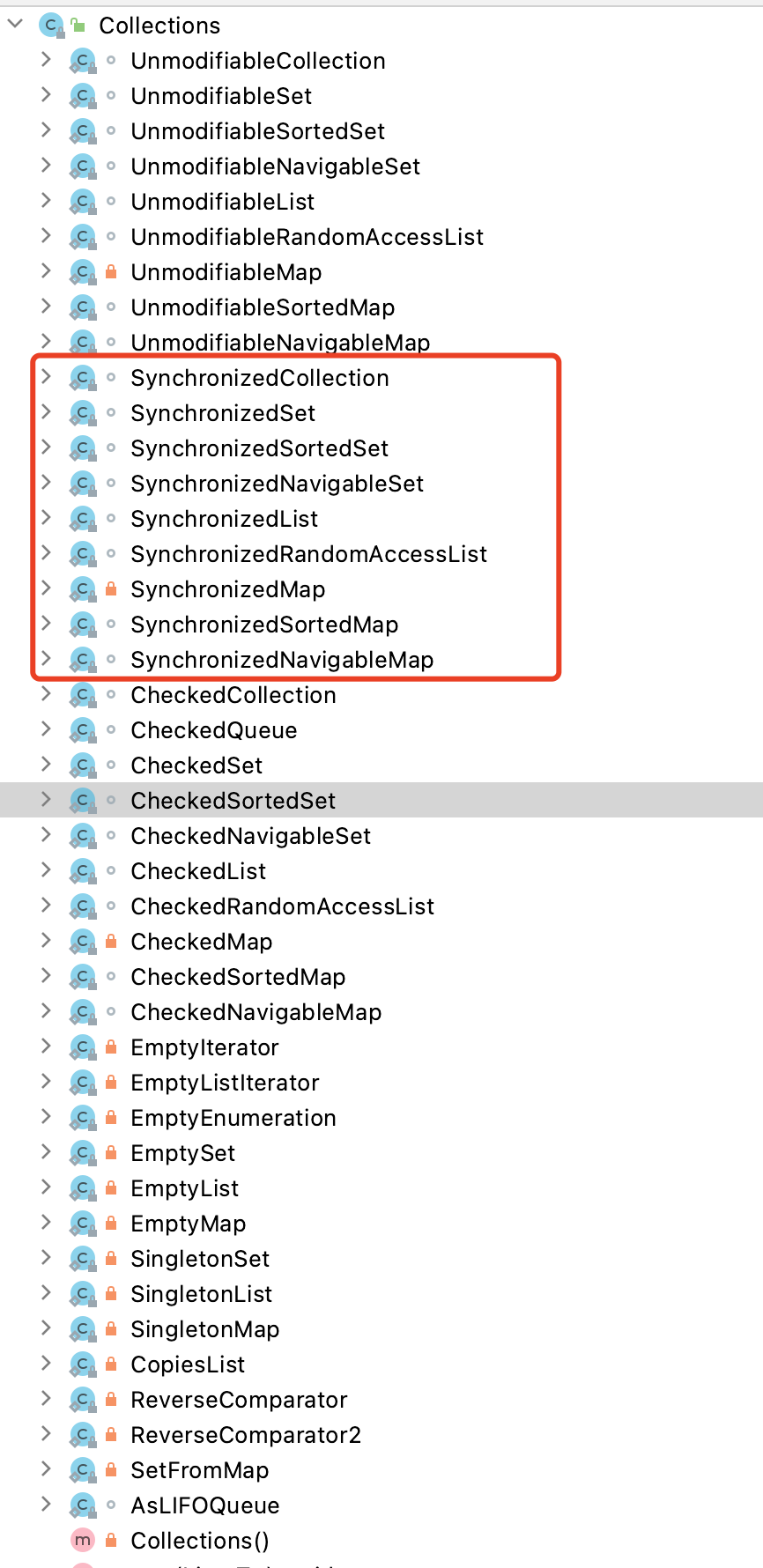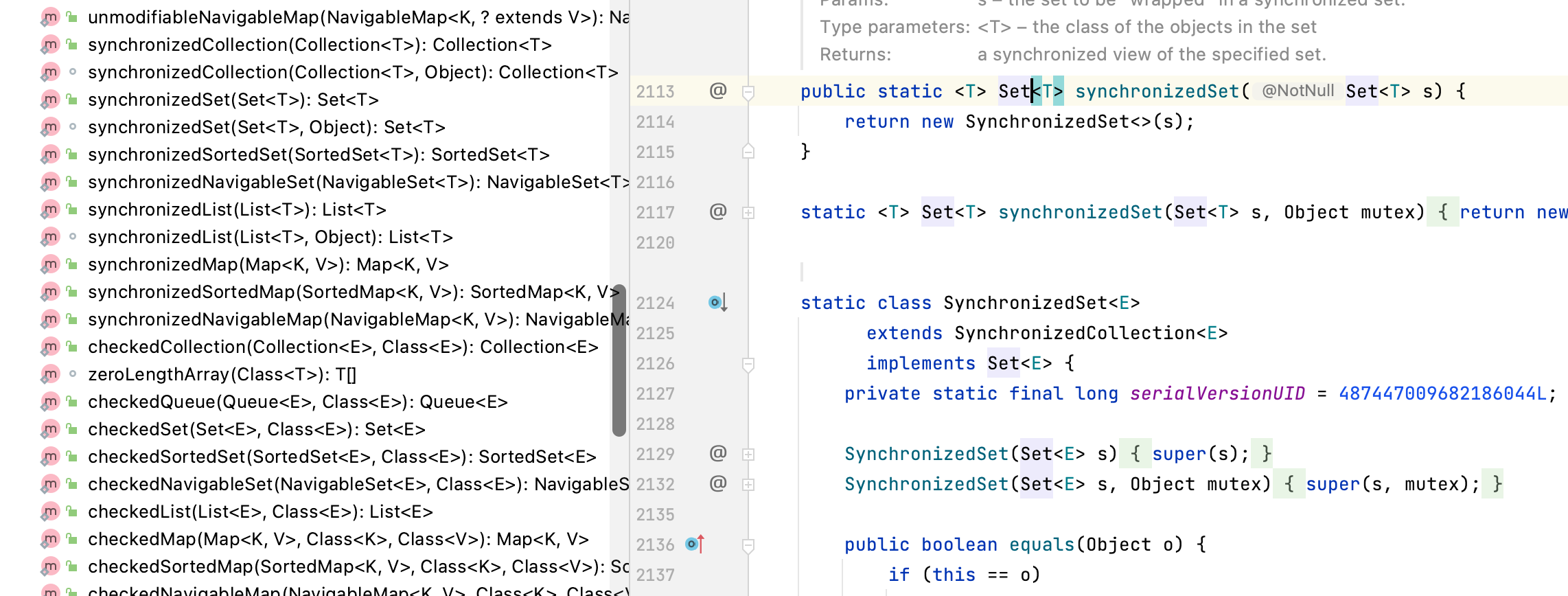``````static <T> Collection<T> synchronizedCollection(Collection<T> c)
static <T> List<T> synchronizedList(List<T> list)
static <K,V> Map<K,V> synchronizedMap(Map<K,V> m)
static <T> Set<T> synchronizedSet(Set<T> s)
``````

``````Collection c =
Collections.synchronizedCollection(myCollection);
...
synchronized (c) {
Iterator i = c.iterator();
...
}
``````

# CyclicBarrier

Synchronized 关键字帮助我们协调多个线程对共享资源的访问。

## 构造函数

• 创建一个cyclibarrier具有指定数量等待它的线程的
``````CyclicBarrier(int threads)
``````
• 创建一个CyclicBarrier (int parties，Runnable barrierAction)具有指定数量的线程等待的
``````CyclicBarrier(int parties, Runnable barrierAction)
``````

## 方法

• 举例
``````class CyclicBarrier1 {
static void checkStep(
CyclicBarrier barrier, String step) {
// Do something to prepare the step
try {
barrier.await();
} catch (Exception e) { /** ... */}
}
public static void main(String[] args) {
String[] steps = {"Read the recipe",
"Gather the ingredients",
"Wash hands"};
System.out.println("Preparing everything:");
Runnable allSet = () ->
ExecutorService executor =
CyclicBarrier barrier =
new CyclicBarrier(steps.length, allSet);
for (String step : steps) {
executor.submit(
() -> checkStep(barrier, step)
);
}
executor.shutdown();
}
}
``````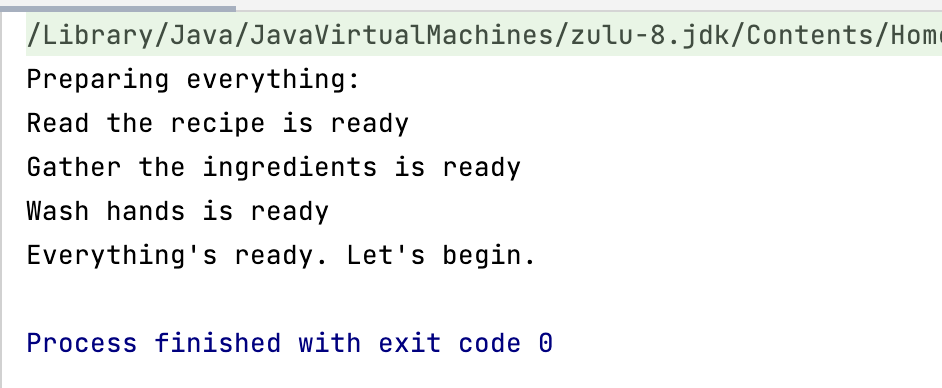# 总结

• 同步与锁一起工作。每个对象都有一个内置锁，因为每个对象只有一个锁，所以任何时候只有一个线程可以持有该锁。通过在块或方法中使用synchronized关键字来获取锁。
• 静态代码也可以同步，但不是使用它来获取类实例的锁，而是在每个类都关联的class获取。
• java.util.concurrent.atomic包包含用于执行原子操作的类（如AtomicInteger），这些操作在一个步骤中执行，不需要超过个线程的干预。
• BlockingQueue表示线程安全队列，BlockingDeque表示线程安全队列。如果队列/队列为空，则等待插入元素（可选超时），如果队列/队列已满，则等待删除元素。
• ConcurrentMap表示线程安全映射，它由ConcurrentHashMap类实现。
• ConcurrentNavigableMap表示线程安全的NavigableMap（如TreeMap），由ConcurrentSkipListMap类实现。